# AP Calculus AB Practice Test 13

### Test Information10 questions20 minutes

Calculator Disallowed

1. The average value of sec2x on the interval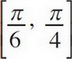is

2. Find the area of the region bounded by the parabolas y = x2 and y = 6x - x2.

3. The function f is given by f(x) = x4 + 4x3. On which of the following intervals is f decreasing?

4.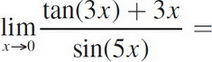5. If the region enclosed by the y-axis, the curve y =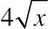, and the line y = 8 is revolved about the x-axis, the volume of the solid generated is

6. The maximum velocity attained on the interval 0 ≤ t ≤ 5, by the particle whose displacement is given by s(t) = 2t3 - 12t2 + 16t + 2 is

7. The value of c that satisfies the Mean Value Theorem for derivatives on the interval [0, 5] for the function f(x) = x3 - 6x is

8. If f(x) = sec (4x), then f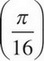is

9.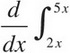cost dt =

10.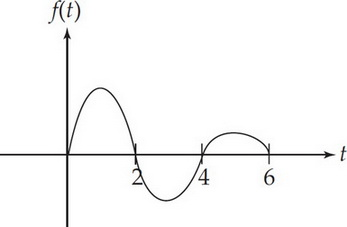Let g(x) =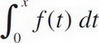where f(t) has the graph shown above. Which of the following could be the graph of g ?